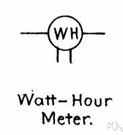# watt-hour

(redirected from Megawatthours)
Also found in: Thesaurus, Encyclopedia.

## watt-hour

(wŏt′our′)
n. Abbr. WH or W-hr
A unit of energy, especially electrical energy, equal to the work done by one watt acting for one hour and equivalent to 3,600 joules.

## watt-hour

n
(Units) a unit of energy equal to a power of one watt operating for one hour. 1 watt-hour equals 3600 joules
Collins English Dictionary – Complete and Unabridged, 12th Edition 2014 © HarperCollins Publishers 1991, 1994, 1998, 2000, 2003, 2006, 2007, 2009, 2011, 2014

## or watt′hour`,

n.
a unit of energy equal to the energy of one watt operating for one hour, equivalent to 3600 joules. Abbr.: Wh

## watt-hour

A unit of power, especially electrical power, equal to the work done by one watt acting for one hour. It is equivalent to 3,600 joules.
 Noun 1watt-hour - a unit of energy equal to the power of one watt operating for one hourenergy unit, heat unit, work unit - a unit of measurement for workB.T.U., Board of Trade unit, kilowatt hour, kW-hr - a unit of energy equal to the work done by a power of 1000 watts operating for one hour